Next: 1.5.6 Parametrized self-organizing maps Up: 1.5 Learning of sensorimotor Previous: 1.5.4 Recurrent neural networks

## 1.5.5 Self-organizing maps

To learn sensorimotor relations, Ritter et al. (1990) used the self-organizing map (SOM) algorithm (Kohonen, 1995). This algorithm fits a q-dimensional grid to a distribution of training patterns in IRd, q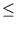d (Kohonen, 1982). SOMs were motivated by sensory maps in the brain, for example, the somatosensory map or the tonotopic map (Kohonen, 1989). A SOM consists of a q-dimensional array1.8 of nodes; each node i has a location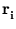and a weight vector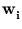(figure 1.7). The weight vectors are in the space of the training patterns.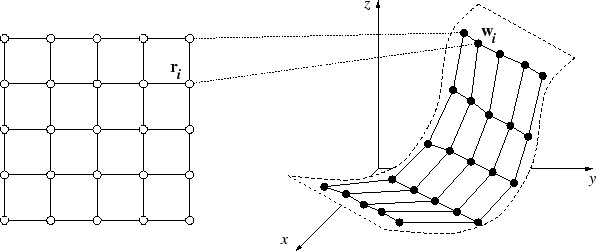The algorithm consists of three steps, which are alternated until convergence is reached. First, a training pattern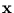is drawn randomly. Second, the node c is determined whose weight vector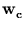is closest to,

 c = arg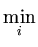||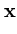-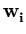|| . (1.2)

Third, all weights are updated, depending on each nodes distance ||-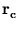|| to the best matching node c,(t + 1) =(t) + hic [(t) -(t)]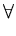i . (1.3)

The neighborhood function hic is

 hic =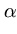(t)exp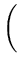-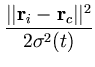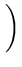. (1.4)

The learning rate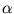(t) and the radius of the neighborhood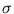(t) both decrease monotonically in time t. The neighborhood function ensures that the grid of weights has the same topology as the array of nodes. If the training patterns lie on a q-dimensional manifold, the algorithm makes the grid of weights to follow this manifold (figure 1.7, right). Many examples can be found in Kohonen (1995).

The SOM algorithm can be easily extended by adding more parameters to each node and by updating them in parallel to the weight vectors. With such an extension, Ritter and Schulten (1986) used the SOM to learn sensorimotor relations. Here, a training pattern consists of a pair (,y) of sensor valuesand corresponding motor values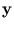. Such a sensorimotor pattern is an element in the space formed by the Cartesian product of the sensor and the motor space. The SOM extension has two weight vectors for each node i, one for the sensory input,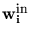, and one for the motor output,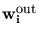. The computation (1.2) of the best matching node is restricted to the sensory domain; but bothandare updated according to (1.3) (is updated based on, and the neighborhood function may have different parameters).

This algorithm fits the grid of weight vectors (,) to the sensorimotor pattern distribution. The resulting link between a sensory inputand a motor outputprovides a discrete mapping, usable for an inverse model. To obtain a continuous mapping, Ritter et al. (1989) further added a locally linear map to each node. The result was successfully applied to control a robot arm with three degrees of freedom.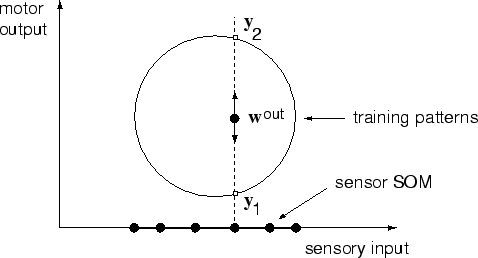The restriction of the node competition (1.2) to the distance in sensory space reduces the search space for the weights, but it makes the approach fail on one-to-many mappings (figure 1.8). The learning foris independent of. As a result, a classical SOM algorithm is applied solely to the sensory domain, whileis updated simultaneously. In the case of two possible target values for one sensory input,is attracted to two different positions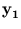and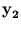(because the node competition is independent of the distance toor). Thus, as a result from the update rule (1.3),will be averaged amongand. For example, given that bothandare drawn with the same probability (p = 0.5), on average,is updated according to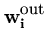(t + 1) =(t) +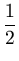hic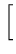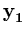-(t)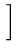+hic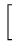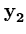-(t)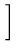=(t) + hic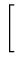(+) -(t)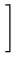. (1.5)

Therefore, the resulting relation between sensory input and motor output is invalid.

Further limitations arise from the SOM grid structure. First, since sensorimotor manifolds are usually non-linear, many grid points are needed. With increasing dimensionality q of the manifold, the number of necessary points increases exponentially (the number of points per dimension to the power of q). Soon (q > 3), this gets computationally unfeasible. As a solution to this problem, Martinetz and Schulten (1990) suggested an extension to hierarchical SOM. A second limitation arises in real world applications: some sensor values could be pure noise (or irrelevant to the sensorimotor map). Such noise dimensions need to be filled with grid points (figure 1.9), resulting in the same problems as mentioned above.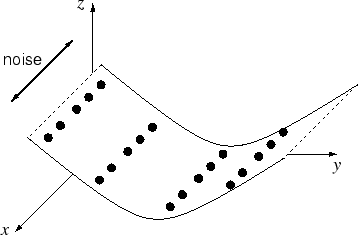Next: 1.5.6 Parametrized self-organizing maps Up: 1.5 Learning of sensorimotor Previous: 1.5.4 Recurrent neural networks
Heiko Hoffmann
2005-03-22